﻿ JavaScript Operators- TutorialsTrend
This Angular tutorial helps you get started with Angular quickly and effectively through many practical examples.
`

# JavaScript Operators

#### JavaScript Operators

An operator performs some operation on single or multiple operands (data value) and produces a result. Operators are symbols or keywords that instruct the JavaScript engine to take certain actions. The addition (+) sign, for example, instructs the JavaScript engine to add two variables or values, whereas the equal-to (=), greater-than (>), or less-than (<) symbols instruct the JavaScript engine to compare two variables or values, and so on.

Let us take a simple expression 3 + 5 is equal to 8. Here, 3 and 5 are called operands and "+" is called the operator.JavaScript contains both unary (single operand) and binary operators (two operands).

Unary operator - Operand operator or Operator operand

Example

a++ or ++a

Binary operator -Operand operator operand

Example

a * b

The following operators are available in JavaScript.

1. Arithmetic Operators
2. Assignment Operators
3. Comparison (Relational) Operators
4. Bitwise Operators
5. Logical Operators
6. String Operator

#### 1. Arithmetic Operators

Arithmetic Operators are used to perform arithmetic on numbers or operands.

The following table show the list of arithmatic operators in JavaScript.

SNo. Operator Type Description with Example
1 Addition (+) Binary It is used to return the value after addition. ex- (20 + 30)
2 Subtraction (-) Binary It is used to return the value after subtraction. ex- (30 - 20)
3 Multiplication (*) Binary It is used to return the value after multiplying the operands. ex- (30 * 20)
4 Division (/) Binary It is used to return the value after dividing 2 operands. ex- (30 / 3)
5 Remainder (%) Binary It is used to return the integer remainder after dividing 2 operands. ex- (30 % 4)
6 Increment (++) Unary It adds 1 to the operand. For the prefix operator (++var2), it returns the value after adding 1. For the postfix operator (var2++), it adds 1 to the value then returns it. ex- 7++
7 Decrement (--) Unary It subtracts 1 from the operand. For the prefix operator (–var2), it returns the value after subtracting 1. For the postfix operator (var2–), it subtracts 1 from the value then returns it. ex- 7--
8 Unary negation (-) Unary It is used to return the negation of the value. ex- +7
9 Unary plus (+) Unary It is used to converts the operand to a number. ex: -7
10 Exponentiation operator (**) Binary It calculates the base to the exponent power, i.e., baseexponent. ex - (4 ** 4)

You can paste the below code in JavaScript editor and run the program.

``````<!DOCTYPE html>
<html>
<meta charset="utf-8" />
<title></title>
<html>
<body>
<h2>TutorialsTrend: Arithmetic Operators Example</h2>
<script>
document.write("20 + 30 = " + (20 + 30) + ".</br>");
document.write("<b>Subtraction:</b>");
document.write("12 - 3 =" + (30 - 20) + ".</br>");
document.write("<b>Multiplication:</b>");
document.write("12 * 3 =" + (30 * 20) + ".</br>");
document.write("<b>Division:</b>");
document.write("12 / 3 =" + (30 / 3) + ".</br>");
document.write("<b>Remainder:</b>");
document.write("12 % 3 =" + (30 % 4) + ".</br>");
var arthvar = 7;
document.write("Value of arthvar is " + + ".</br>");
document.write("<b>Increment:</b></br>");
document.write("arthvar++ is " + (arthvar++) + ". ");
document.write("Value of arthvar is " + arthvar + ".</br>");
document.write("++arthvar is " + (++arthvar) + ". ");
document.write("Value of arthvar is " + arthvar + ".</br>");
document.write("<b>Decrement:</b></br>");
document.write("arthvar-- is " + (arthvar--) + ". ");
document.write("Value of arthvar is " + arthvar + ".</br>");
document.write("--arthvar is " + (--arthvar) + ". ");
document.write("Value of arthvar is " + arthvar + ".</br>");
document.write("<b>Unary negation:</b></br>");
document.write("-arthvar is " + (-arthvar) + ".</br>");
document.write("<b>Unary plus:</b></br>");
document.write("+arthvar is " + (+arthvar) + ".</br>");
document.write("+true is " + (+true) + ".</br>");
document.write("<b>Exponentiation operator:</b>");
document.write("4 ** 4 is " + (4 ** 4) + ".</br>");
</script>
</body>
</html>
<body>
</body>
</html>``````

Output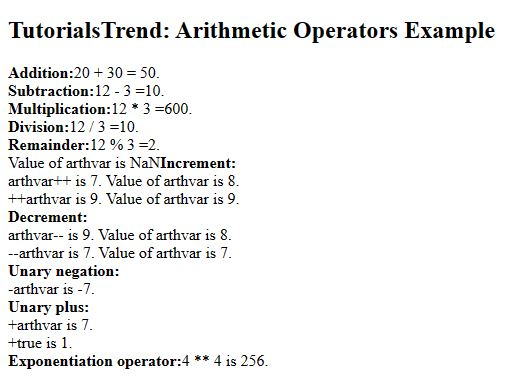#### 2. Assignment Operators

An assignment operator is used to assigns a value to the left operand based on the value of its right operand with the help of equals = sign.

The following table show the list of Assignment operators in JavaScript.

SNo. Operator Description Shorthand Meaning
1 = Assignment x = y x = y
2 += Add and Assignment x += y x = x + y
3 -= Subtract and Assignment x -= y x = x - y
4 *= Multiply and Assignment x *= y x = x * y
5 /= Division Assignment x /= y x = x / y
6 %= Remainder Assignment x %= y x = x % y
7 **= Exponentiation Assignment x **= y x = x ** y

You can paste the below code in JavaScript editor and run the program.

``````<!DOCTYPE html>
<html lang="en">
<meta charset="utf-8">
<title>TutorialsTrend - JavaScript Assignment Operators</title>
<body>

<h2>TutorialsTrend: Arithmetic Assignment Example</h2>
<script>
var a;
a = 10;
document.write("= Operator =" + a + "<br>");

a = 20;
a += 30;
document.write("+= Operator=" + a + "<br>");

a = 50;
a -= 20;
document.write("-= Operator=" + a + "<br>");

a = 5;
a *= 25;
document.write("*= Operator=" + a + "<br>");

a = 50;
a /= 10;
document.write("/= Operator=" + a + "<br>");

a = 100;
a %= 15;
document.write("%= Operator=" + a + "<br>");

a = 100;
a **= 15;
document.write("**= Operator=" + a);

</script>
</body>
</html>``````

Output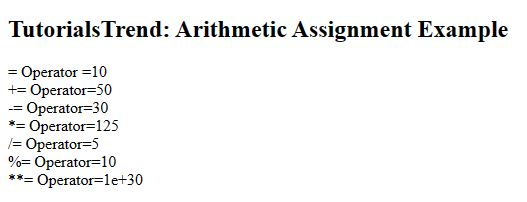#### 3. Comparison Operators

A comparison operator compares its operands and returns a boolean value (true or false) based on whether the comparison is true.

The following table show the list of Comparison operators in JavaScript.

SNo. Operator Name Example Description
1 == Equal x == y True if x is equal to y
2 === Identical x === y True if x is equal to y, and they are of the same type
3 != Not equal x != y True if x is not equal to y
4 !== Not identical x !== y True if x is not equal to y, or they are not of the same type
5 < Less than x < y True if x is less than y
6 > Greater than x > y True if x is greater than y
7 >= Greater than or equal to x >= y True if x is greater than or equal to y
8 <= Less than or equal to x <= y True if x is less than or equal to y

You can paste the below code in JavaScript editor and run the program.

``````<!DOCTYPE html>
<html lang="en">
<meta charset="utf-8">
<title>TutorialsTrend - JavaScript Comparison Operators</title>
<body>

<h2>TutorialsTrend - JavaScript Comparison Operators</h2>

<script>
var x = 35;
var y = 45;
var z = "35";

document.write("== Operator : ");
document.write(x == z);
document.write("<br>");

document.write("=== Operator : ");
document.write(x === z);
document.write("<br>");

document.write(" != Operator : ");
document.write(x != y);
document.write("<br>");

document.write(" !== Operator : ");
document.write(x !== z);
document.write("<br>");

document.write(" < Operator : ");
document.write(x < y);
document.write("<br>");

document.write(" > Operator : ");
document.write(x > y);
document.write("<br>");

document.write(" <= Operator : ");
document.write(x <= y);
document.write("<br>");

document.write(" >= Operator : ");
document.write(x >= y);
</script>
</body>
</html>``````

Output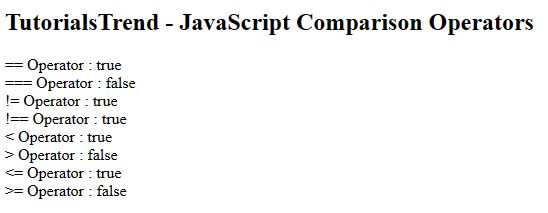#### 4. Bitwise Operators

The bitwise operators perform bitwise operations on operands. The bitwise operators are as follows:

SNo. OperatorNameExampleDescription
1&Bitwise AND(10==20 & 20==33) = 0It returns 1 if both operands are ones
2|Bitwise OR(10==20 | 20==33) = 0It returns 0 if both operands are zero
3^Bitwise XOR(10==20 ^ 20==33) = 0It returns 0 in each bit position for which the corresponding bits are the same and 1 in each bit position for which the corresponding bits are different.
4~Bitwise NOT (~10) = -11The operand's bits are inverted by it.
5<<Bitwise Left Shift(10<<2) = 40It shifts x in binary representation y bits to the left, shifting in zeros from the right.
6>>Bitwise Right Shift(10>>2) = 2It shifts x in binary representation y bits to the right, discarding bits shifted off.
7>>>Bitwise Right Shift with Zero(10>>>2) = 2It shifts x in binary representation y bits to the right, discarding bits shifted off.

You can paste the below code in JavaScript editor and run the program.

``````<!DOCTYPE html>
<html lang="en">
<meta charset="utf-8">
<title>TutorialsTrend - JavaScript Bitwise Operators</title>
<body>
<h2>TutorialsTrend - JavaScript Bitwise Operators</h2>

<script>
document.write(10==20 & 20==33);
document.write("<br>");

document.write(10==20 | 20==33);
document.write("<br>");

document.write(10==20 ^ 20==33);
document.write("<br>");

document.write(~10);
document.write("<br>");

document.write(10<<2);
document.write("<br>");

document.write(10>>2);
document.write("<br>");

document.write(10>>>2);
document.write("<br>");
</script>
</body>
</html>``````

Output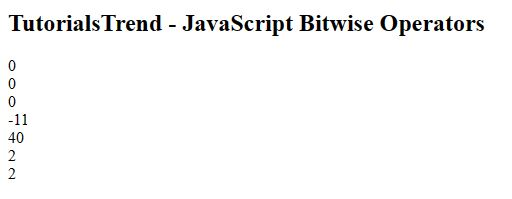#### 5. Logical Operators

These operators are (typically) used with Boolean/ logical values and return a Boolean value. However, if the && and || operators are used with non-Boolean values, they may return a non-Boolean value.

The following table show the list of Logical operators in JavaScript.

SNo.OperatorNameExampleDescription
1 `&&` And `x && y` True if both x and y are true
2 `||` Or `x || y` True if either x or y is true
3 `!` Not `!x` True if x is not true

You can paste the below code in JavaScript editor and run the program.

``````<!DOCTYPE html>
<html lang="en">
<meta charset="utf-8">
<title>TutorialsTrend - JavaScript Logical Operators</title>
<body>
<h2>TutorialsTrend - JavaScript Logical Operators</h2>
<script>
var x = 8;
var y = 3;
document.write("<b>Logical AND:</b></br>");
document.write(x < 10 && y > 1);
document.write("<br>");
document.write(x < 10 && y < 1);

document.write("<br>");
document.write("<b>Logical OR:</b></br>");
document.write(x < 10 || y > 1);
document.write("<br>");
document.write(x < 10 || y < 1);

document.write("<br>");
document.write("<b>Logical NOT:</b></br>");
document.write(!(x === y));
document.write("<br>");
document.write(!(x > y));
</script>
</body>
</html>``````

Output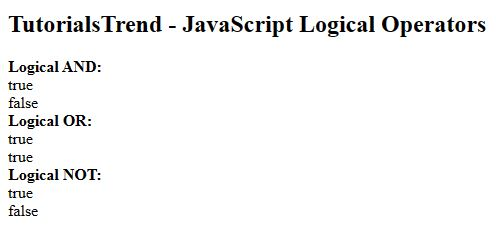#### 6. String Operator

Sometimes you need to join two or more strings together in JavaScript. Technically, joining two strings is known as string concatenation. We use the concatenation operator (+) for this purpose.

The following table show the list of String operators in JavaScript.

SNo.OperatorNameExampleDescription
1 + Concatenation string1 + string2 Concatenation of string1 and string2
2 += Concatenation assignment string1 += string2 Appends the string2 to the string1

You can paste the below code in JavaScript editor and run the program.

``````<!DOCTYPE html>
<html lang="en">
<meta charset="utf-8">
<title>TutorialsTrend - JavaScript String Operators</title>
<body>
<h2>TutorialsTrend - JavaScript String Operators</h2>
<script>
var string1 = "Tutorials";
var string2 = " Trend!";
document.write(string1 + string2 + "<br>");